+关注继续查看

# 前言

## 1.3 欠拟合和过拟合

### 1.3.1 模型复杂度k## 1.4 多项式函数拟合示例

%matplotlib inline
import torch
import numpy as np
import sys
import d2lzh_pytorch as d2l


### 1.4.1 生成数据集n_train, n_test, true_w, true_b = 100, 100, [1.2, -3.4, 5.6], 5
# torch.randn:用来生成随机数字的tensor，这些随机数字满足标准正态分布（0~1）
features = torch.randn((n_train + n_test, 1))
# torch.cat()把多个tensor进行拼接，poly_features.shape=200*3
poly_features = torch.cat((features, torch.pow(features, 2), torch.pow(features, 3)), 1)
labels = (true_w * poly_features[:, 0] + true_w * poly_features[:, 1]
+ true_w * poly_features[:, 2] + true_b)
# np.random.normal()的意思是一个正态分布,噪声
labels += torch.tensor(np.random.normal(0, 0.01, size=labels.size()), dtype=torch.float)


features[:2], poly_features[:2], labels[:2]
? 1

(tensor([[-1.0613],
[-0.8386]]), tensor([[-1.0613,  1.1264, -1.1954],
[-0.8386,  0.7032, -0.5897]]), tensor([-6.8037, -1.7054]))


### 1.4.2 定义、训练和测试模型

def semilogy(x_vals, y_vals, x_label, y_label, x2_vals=None, y2_vals=None,
legend=None, figsize=(3.5, 2.5)):
d2l.set_figsize(figsize)
d2l.plt.xlabel(x_label)
d2l.plt.ylabel(y_label)
d2l.plt.semilogy(x_vals, y_vals)
if x2_vals and y2_vals:
d2l.plt.semilogy(x2_vals, y2_vals, linestyle=':')
d2l.plt.legend(legend)


num_epochs, loss = 100, torch.nn.MSELoss()

def fit_and_plot(train_features, test_features, train_labels, test_labels):
net = torch.nn.Linear(train_features.shape[-1], 1)
# 通过Linear文档可知，pytorch已经将参数初始化了，所以我们这里就不手动初始化了

batch_size = min(10, train_labels.shape)

dataset = torch.utils.data.TensorDataset(train_features, train_labels)
optimizer = torch.optim.SGD(net.parameters(), lr=0.01)

train_ls, test_ls = [], []
for _ in range(num_epochs):
for X, y in train_iter:
l = loss(net(X), y.view(-1, 1))
l.backward()
optimizer.step()
train_labels = train_labels.view(-1, 1)
test_labels = test_labels.view(-1, 1)
train_ls.append(loss(net(train_features), train_labels).item())
test_ls.append(loss(net(test_features), test_labels).item())

print('final epoch: train loss', train_ls[-1], 'test loss', test_ls[-1])
semilogy(range(1, num_epochs + 1), train_ls, 'epochs', 'loss',
range(1, num_epochs + 1), test_ls, ['train', 'test'])
print('weight:', net.weight.data,
'\nbias:', net.bias.data)


### 1.4.3 三阶多项式函数拟合（正常）

fit_and_plot(poly_features[:n_train, :], poly_features[n_train:, :],
labels[:n_train], labels[n_train:])


final epoch: train loss 0.00010175639908993617 test loss 9.790256444830447e-05
weight: tensor([[ 1.1982, -3.3992,  5.6002]])
bias: tensor([5.0014])### 1.4.4 线性函数拟合（欠拟合）

fit_and_plot(features[:n_train, :], features[n_train:, :], labels[:n_train],
labels[n_train:])


final epoch: train loss 249.35157775878906 test loss 168.37705993652344
weight: tensor([[19.4123]])
bias: tensor([0.5805])### 1.4.5 训练样本不足（过拟合）

fit_and_plot(poly_features[0:2, :], poly_features[n_train:, :], labels[0:2],
labels[n_train:])


final epoch: train loss 1.198514699935913 test loss 166.037109375
weight: tensor([[1.4741, 2.1198, 2.5674]])
bias: tensor([3.1207])# 总结

• 由于无法从训练误差估计泛化误差，一味地降低训练误差并不意味着泛化误差一定会降低。机器学习模型应关注降低泛化误差。
• 可以使用验证数据集来进行模型选择。
• 欠拟合指模型无法得到较低的训练误差，过拟合指模型的训练误差远小于它在测试数据集上的误差。
• 应选择复杂度合适的模型并避免使用过少的训练样本。# 【深度学习】：《100天一起学习PyTorch》模型评估和选择（上）：欠拟合和过拟合

50 0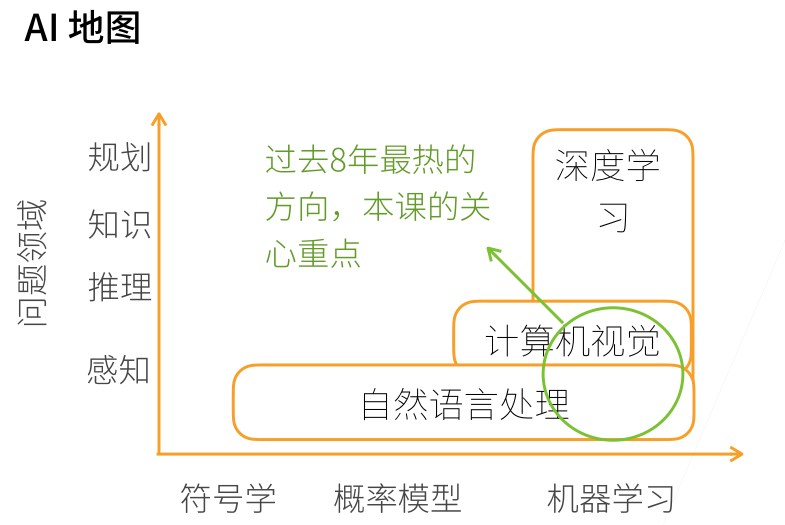《动手学深度学习》学习随笔
《动手学深度学习》学习随笔
8 0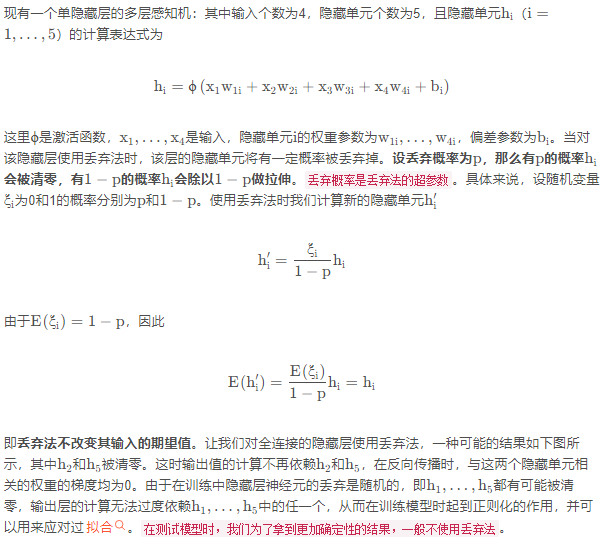【从零开始学习深度学习】14. 防止过拟合方法：Dropout方法介绍及示例演示
【从零开始学习深度学习】14. 防止过拟合方法：Dropout方法介绍及示例演示
41 0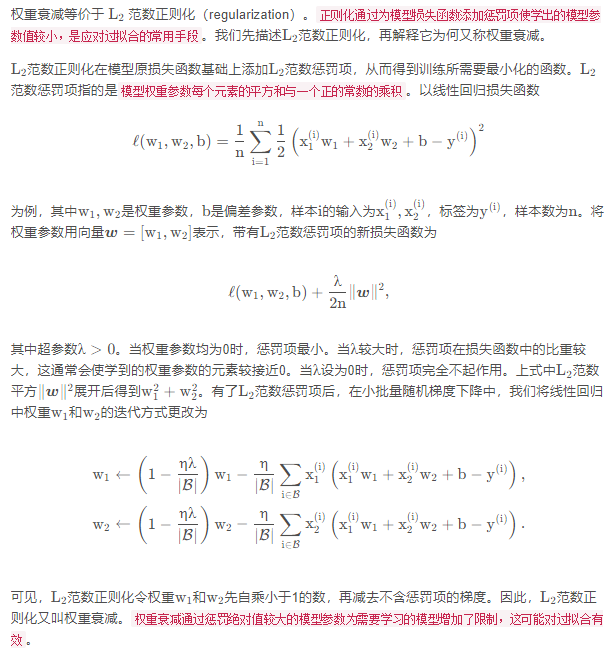【从零开始学习深度学习】13. 防止过拟合方法：权重衰减(L2惩罚项)介绍及示例演示
【从零开始学习深度学习】13. 防止过拟合方法：权重衰减(L2惩罚项)介绍及示例演示
30 0【从零开始学习深度学习】11.使用Pytorch实现多层感知机的构建与训练
【从零开始学习深度学习】11.使用Pytorch实现多层感知机的构建与训练
45 0【从零开始学习深度学习】10.自己动手从零开始实现一个多层感知机
【从零开始学习深度学习】10.自己动手从零开始实现一个多层感知机
27 0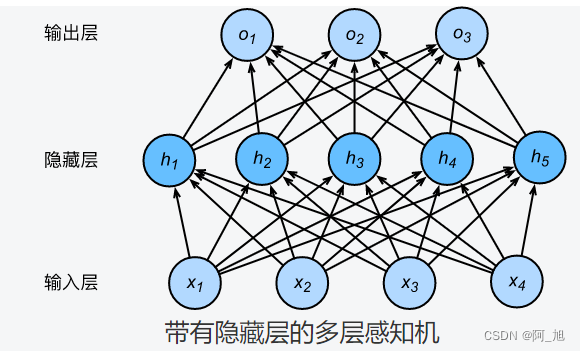【从零开始学习深度学习】9.多层感知机MLP及常用激活函数介绍
【从零开始学习深度学习】9.多层感知机MLP及常用激活函数介绍
54 0【从零开始学习深度学习】8.Pytorch实现softmax回归模型训练
【从零开始学习深度学习】8.Pytorch实现softmax回归模型训练
56 0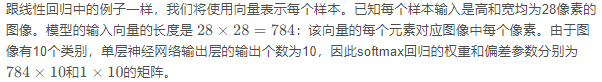【从零开始学习深度学习】7.自己动手实现softmax回归的训练与预测
【从零开始学习深度学习】7.自己动手实现softmax回归的训练与预测
52 02996121712224591

【算法实战】9. 线性回归算法325

【算法实战】5. Logistic回归算法396

【算法实战】4. 朴素贝叶斯算法996

TensorRT Introduction